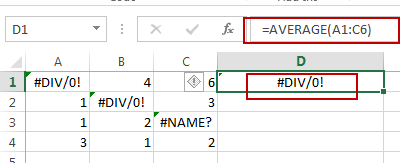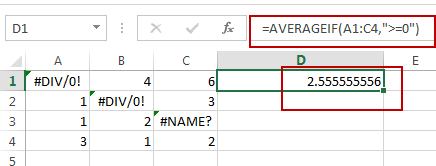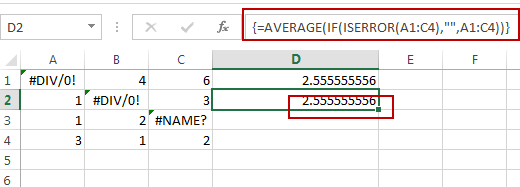# How to Ignore Error Values When Calculating the Average in Excel

This post will guide you how to ignore error values when getting average for a range of cells in Excel. How do I average a range of cells ignoring error values, such as: #div/0!.

Assuming that you have a list of range of cells that you want to calculate the average of those cells which including error values , such as: #div/0 or #NAME?, etc. When you use the Average function to calculate those cells, you will get an error result. So How to handle this in Excel.If you want to ignoring any errors that might exist in the given range, you can use AVERAGEIF function or AVERAGE function with IF function to achieve the result. Like this:

`=AVERAGEIF(A1:C4,”>=0”)`

Or

`=AVERAGE(IF(ISERROR(A1:C4),””,A1:C4))`

OR

`=AVERAGE(IF(ISNUMBER(A1:C4),A1:C4))`Note: The second and third formula need to press Ctrl + Shift +  Enter keys, as those formulas are Array Formula. A1:C4 is the data range that you wish to average. And you need to change it as you need.

Let’s see how the first formula works: the AVERAGEIF function can be used to calculate an average of numeric values with one or more criteria for a given range. And In this example, the criteria is the expression “>=0”, it will filter out all error values, and just to calculate the positive numeric values in the given range.

### Related Functions

• Excel IF function
The Excel IF function perform a logical test to return one value if the condition is TRUE and return another value if the condition is FALSE. The IF function is a build-in function in Microsoft Excel and it is categorized as a Logical Function.The syntax of the IF function is as below:= IF (condition, [true_value], [false_value])….
• Excel AVERAGE function
The Excel AVERAGE function returns the average of the numbers that you provided.The syntax of the AVERAGE function is as below:=AVERAGE (number1,[number2],…)….
• Excel AVERAGEIF function
The Excel AVERAGEAIF function returns the average of all numbers in a range of cells that meet a given criteria.The syntax of the AVERAGEIF function is as below:= AVERAGEIF (range, criteria, [average_range])….
• Excel IFERROR function
The Excel IFERROR function returns an alternate value you specify if a formula results in an error, or returns the result of the formula.The syntax of the IFERROR function is as below:= IFERROR (value, value_if_error)….
• Excel ISNUMBER function
The Excel ISNUMBER function returns TRUE if the value in a cell is a numeric value, otherwise it will return FALSE.The syntax of the ISNUMBER function is as below:= ISNUMBER (value)…
Related Posts

Count Cells that are Case Sensitive

If you are a frequent user of Microsoft Excel, you may have come across situations where you needed to add or count the cells that were case-sensitive. If you have done this task manually, I would like to point out ...

Abbreviate Names Or Words in Excel

As an MS Excel user, you might have come across a task where you need to abbreviate different names or words, and there are also possibilities that you might have done this task manually by assuming that there isn't any ...

Filter or Remove Columns

Assume that in MS Excel, you have a table consisting of a few columns consisting of few values, and you want to filter to remove the specified columns from the table. You might take it easy and would prefer to ...

Filter or Extract for Multiple OR Criteria

If you are an valid MS Excel user, you have probably come across a situation where you wanted to filter the data in a separate table with specific criteria. You could do this task manually, which is also acceptable when ...

Filter or Extract with a Partial Match

Assume you have a table consisting of a few cells having few values, and you want to filter out the set of records with the partial match. You might take it easy and would prefer to manually filter out the ...

Filter Multiple Values

In Excel, you can easily filter a table to display only the rows that meet your criteria. This is a quick way to find the information you need without scrolling through all the data. In this post, we'll show you ...

Extract all Partial Matches

Just assume that you have a range of data that contains a text string and you want to extract the few partial matches into another separate range of cells; then you might think that it's not a big deal; because ...

If Cell Contains Certain Text OR Equals Certain Text

IF cell equals certain text IF function is frequently used in Excel worksheet to return “true value” or “false value” based on the logical test result. If you want to test values to see if they equal certain text like ...

Average the Last N Numeric Values in Excel

AVERAGE function is one of the most popular functions in Excel. Apply AVERAGE together with some other functions, we can calculate average simply for some complex situations. In this article, we will introduce you to calculate average of the last ...

How to Count Cells that Contain only numbers in Excel

This post will guide you how to count the number of cells that only contain numbers within a range of cells using a formula in Excel 2013/2016. Count Number of Cells that Contain Numbers Assuming that you have a data ...

Sidebar